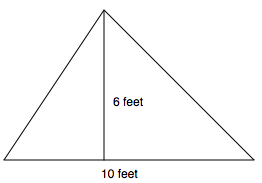### Home > MC1 > Chapter 9 > Lesson 9.3.2 > Problem9-139

9-139.

Calculate the area of each triangle described below.

1. Base: $10$ feet, Height: $6$ feet

The area of a triangle is equal to $\left(\frac{1}{2}\right)(\text{base})(\text{height})$.$\left(\frac{1}{2}\right)(10)(6)=30\text{ feet}^2$

2. Base: $14$ mm, Height: $5$ mm

Draw a diagram like the one in part (a), if you need help visualizing the triangle.